## Artigo

•Citado por SciELO
•Acessos

•Citado por Google
•Similares em SciELO
•Similares em Google

## versão impressa ISSN 0120-100X

### Rev. ion vol.27 no.1 Bucaramanga jan./jun. 2014

Determination of increased mean drag coefficients for a cylinder
vibrating at low values of Keulegan-Carpenter number

Carlos Alberto Riveros1*; Edwin Fabián García2; Javier Enrique Rivero2

1 Programa de Ingeniería Oceanográfica, Escuela Ambiental, Facultad de Ingeniería,
*riveros@udea.edu.co
2 Programa de Ingeniería Civil, Escuela Ambiental, Facultad de Ingeniería, Universidad de Antioquia,

Fecha Recepción: 13 de agosto de 2013
Fecha Aceptación: 19 de febrero de 2014

Abstract

There is an increasing demand for the development of a reliable technology for wind turbines in deep-waters. Therefore, offshore wind turbine technology is receiving great amount of attention by the research community. Nevertheless, the dynamic response prediction of the support system for offshore wind turbines is still challenging due to the nonlinear and self-regulated nature of the Vortex Induced Vibration (VIV) process. In this paper, the numerical implementation of a computational fluid dynamics-based approach for determination of increased mean drag coefficient is presented. The numerical study is conducted at low values of Keulegan-Carpenter number in order to predict the increment of drag force due to cross-flow motion. The simulation results are then compared with previously developed empirical formulations. Good agreement is observed in these comparisons.

Keywords: computational fluid dynamics, vortex-induced vibration, drag coefficient; inertia coefficient, Keulegan-Carpenter number, cross-flow motion.

Determinación de coeficientes de arrastre aumentados para un
cilindro que vibra con valores bajos del número Keulegan-Carpenter

Resumen

Palabras Clave: dinámica de fluidos computacional, vibración inducida por vórtices, coeficiente de arrastre, coeficiente de inercia, número de Keulegan-Carpenter, vibración transversal al flujo.

Determinação dos coeficientes de arrasto incrementados para um
cilindro vibrando a baixos valores de número Keulegan-Carpenter

Resumo

Há uma crescente demanda para apoiar o desenvolvimento de uma tecnologia confiável para turbinas eólicas em águas profundas. Portanto, a tecnologia de turbinas eólicas ultramar tem recebido grande atenção por parte da comunidade cientifica. No entanto, a previsão de resposta do sistema de suporte para turbinas eólicas ultramar ainda é um desafio devido à natureza não-linear e auto-regulada do processo de geração de vórtices induzidos por vibração. Este artigo, descreve a implementação numérica de um procedimento baseado na dinâmica computacional de fluidos para determinar os coeficientes de arrasto incrementados. A análise numérica é realizada para valores baixos de números Keulegan-Carpenter, com a finalidade de prever o aumento da força de arrasto, devido ao movimento transversal ao fluxo. Os resultados da simulação são comparados com as formulações empíricas anteriormente desenvolvidas. Bons resultados são observados nessas comparações.

Palavras-chave: dinâmica computacional de fluidos, vibração induzida por vórtices, coeficiente de arrasto, coeficiente de inércia, número de Keulegan - Carpenter, vibração transversal ao fluxo.

Citar como: Riveros CA, García EF, Rivero JE. Determination of increased mean drag coefficients for a cylindervibrating at low values of Keulegan-Carpenter number. rev.ion. 2014;27(1):7-15.

Introduction

Two years after the March 11 earthquake and resultant tsunami that caused a meltdown at the Fukushima Daiichi nuclear plant, the occurrence of future tsunamis that could strike other coastal areas of the world with equal or greater force had changed the perception that nuclear energy is the best option to meet the growing requirement of electricity worldwide. This fact has directed research efforts to search for renewable sources of energy and considering that offshore wind energy and ocean energy together could potentially satisfy all the demands of clean and reliable energy in the long term; many research projects had been proposed in the last two years in order to develop offshore wind farms. In addition, the use of floating wind turbines in deep sea is one of the most promising technologies for generating clean and reliable energy. Recent technology limitations for deep-water construction are mainly related to the lack of reliable and profitable structural systems. The components needed for the development of offshore wind turbines among others include the support system that is based in the use of cables anchored in some cases to sea bed in order to prevent the movement of the wind turbine due to wave motion. Therefore shallow water sites are needed to safely anchor wind turbines, which is not feasible near many major cities. One solution is found in deeper waters where there are no restrictions imposed by generation of noise and commercial shipping, wind force is also more stable leading to a better performance of the energy generation system. However, stabilizing a floating wind turbine in deep waters is a major challenge because of the inherently large mass of the wind turbine and the location of the rotor 80 or 100 meters above sea level. The gravity-based offshore wind turbine is related to the principle of locating its centre of gravity as low as possible. A large floater surface is also used to support offshore wind turbines and consists on a wide area at the free surface which produces large geometrical inertia and then stabilizes the structure. Finally, tension-leg platforms moored to the seabed are also a possible option for stabilization of offshore wind turbines. All these systems are mainly composed of round shape components making it the most widely used geometrical configuration for constructing all the structural components of offshore wind turbines. It is basically due to its inherently symmetry when fluid forces are applied to its surface, tension cables and other support systems are cylindrical and therefore is the most preferred shape for hydrodynamic studies. The aforementioned facts lead to the conclusion that comprehensive understanding of the fluid forces acting on a cylinder is required. Nevertheless, Vortex-Induced Vibration (VIV), commonly associated to the dynamic response of different structural members affected by fluid forces, is a self-regulated process and its accurate prediction is still a matter of research . Therefore, there is an increasing interest in the development of robust dynamic prediction models for VIV. Most of the existing models for VIV prediction rely on experimentally derived hydrodynamic coefficients and although there is an increasing interest in implementing Navier-Stokes solvers for calculation of hydrodynamic forces acting on a structural member immersed in fluid, there are still some limitations related to the computer resources needed for practical applications. The resonant type VIV leads to a considerable increment in the cross-flow amplitude response of a body. This mechanism is usually defined as a lock-in event and its occurrence has been widely reported in experimental studies and field measurements . However, its nonlinear nature is still not well understood. During a lock-in event, the shedding frequency does not follow the Strouhal law leading to a rapid rise of drag and lift forces . At low values of mass and damping, the magnitude of the in-line force experienced by a body which is also moving in the cross-flow direction is different to the in-line force experienced by the same body when fixed conditions are imposed in both directions; drag force experiences an increment when the body freely moves in the cross-flow direction. This paper presents a numerical study considering the case of a freely oscillating cylinder and therefore the increment in the in-line drag force is numerically calculated.

Hydrodynamic behavior of an oscillating body
Oscillatory flow past a stationary cylinder is usually associated to wave motion in sea acting on a body. This fact is true in members located near the sea level where the wave current is stronger. Taking the length of the cylinder as unity, the inline force experienced by a cylinder in oscillatory flow is shown in Eq. 1, the absolute sign is used to take into account the changing direction of the force and the instantaneous velocity U,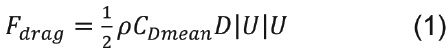where D is the cylinder's diameter and ρ is the fluid density. Although CDmean is a constant parameter which mainly depends on the Reynolds Number (Re), other parameters such as the shape of the body and roughness of the surface are also important. For the case of a circular cylinder across the flow the Re number can be expressed as shown in Eq. 2 using the kinematic viscosity of the fluid defined as μ: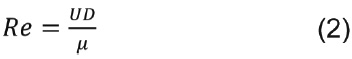For a stationary cylinder excited by oscillatory flow the inertia force can be written using the inertia coefficient, Cm, as shown in Eq. 3: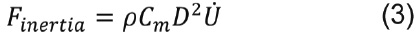Combining the two force components related to Cm and CDmean, the total force per unit length experienced by a stationary cylinder under oscillatory flow, Fosc, is obtained and corresponds to the widely known Morison's equation, which is described by Eq. 4: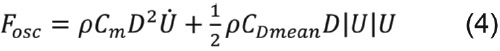Cm and CDmean are functions of Re and Keulegan-Carpenter (KC) numbers. In the case of oscillatory flow past a stationary cylinder Re, KC the β parameter are expressed in terms of the amplitude of the oscillatory motion, U0, and the period of the oscillatory motion, Tf, as shown in the Eq. 5: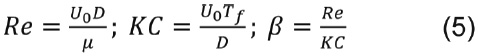However, it is also important to consider the mass of the body and therefore it is possible to assume for large values of mass ratio (mass of a body divided by the mass of the fluid displaced) that the case of an oscillating body in calm fluid is equivalent and kinematically identical to oscillating fluid flowing past a stationary body. As the mass of a body approximates the mass of the fluid that is being displaced by the body, the behaviour drastically changes leading to a more complex response. It is possible to state that the fluid motion is dominant over the response of the cylinder for low values of mass ratio. Hydrodynamic force on an oscillating body at low values of mass ratio includes added mass and damping forces in addition to lift. Furthermore, in the case of an oscillating cylinder the lift force may be substantially different from that measured on an equivalent stationary cylinder. Sarpkaya  made a clear distinction between vortex-shedding excitation and attenuating damping. The latter is associated to an oscillating body in a fluid otherwise at rest and implies a decrease of the amplitude of the externally imparted oscillation by forces in anti-phase with velocity. It is clear that the unseparated flow about the oscillating body does not give rise to oscillatory forces in any direction and, thus, it cannot excite the body. Sarpkaya  highlighted that hydrodynamic damping is still used to lump into one parameter the existing inability to predict the dynamic response of fluid-structure interactions. Added mass and hydrodynamic damping are relevant when are related to bodies with large values of mass ratio. The resulting added mass acting on bodies with large values of mass ratio is very small in comparison with the mass of a body for typical engineering structures and therefore contributes little to the overall response of the system. It is also important to consider that lift forces at resonance are equilibrated by hydrodynamic damping, this signifies that damping force generated on a cylinder having a low mass ratio is of the same magnitude as lift force.

Effect of body motion on wake
Effects on lift force resulting from body motion on the wake include changes in the lift force magnitude, frequency and correlation length. Cross-flow vibration with frequency at or near the vortex shedding frequency organizes the wake. When the body oscillation frequency deviates from the vortex shedding frequency, correlation length drops off quickly towards the values of a stationary body. This facts shows that low values of mass ratio leads to a behaviour much more related to the nature of the fluid acting on the body. Body vibration with frequencies near the vortex shedding influences the pattern and phasing of the vortices. As the body vibration passes through the vortex shedding, there is a clear and rather abrupt 180° phase shift. After the phase shift, shedding of vortices takes place at maximum body cross-flow amplitude. An increment, in transverse amplitude, to values of approximately 1.5D (diameter of the cylinder) leads to break up of the symmetric pattern of alternate vortices. As a result, it is observed that an amplitude of 1.0D, three vortices are formed per cycle of vibration instead of the stable pattern of two per cycle at lower amplitudes. Therefore, this break up implies that the vortex shedding forces applied to the cylinder are a function of cylinder amplitude and may be self-limiting at large vibration amplitudes. It is interesting to note that although the nonlinear nature of the body vibrating near the vortex shedding frequency will not overpass the cross-flow amplitude of approximately 1.5D.

Increased mean drag coefficient
It is sufficiently proved that the average (steady) drag on a cylinder vibrating at or near the vortex shedding frequency is a strong function of its corresponding cross-flow amplitude. Substantial increment in drag can occur for a cylinder vibrating in resonance with vortex shedding. Mean drag coefficient is known to increase two or three times (even four times in some experiments) over the value for stationary cylinder as a result of lock-in . Mean drag amplification was first discovered by Bishop and Hassan . Since then empirical formulas for increased drag, valid for the lock-in region, have been proposed by several investigators (Vandivier , Sarpkaya ). Most of these expressions give similar values (within 15%). Vandivier  proposed an empirical relation for the prediction of drag coefficients under lock-in conditions at Reynolds numbers up to 22000. Vandivier  used data from field experiments and concluded that drag coefficients in excess of 3.0 are possible under lock-in conditions. The empirical relation for increased mean drag coefficient, CDinc, proposed by Vandivier  using the root mean square antinode amplitude, Yrms, is shown in Eq. 6.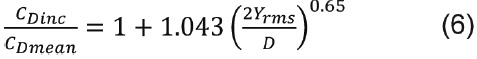Chaplin et al.  showed the validity of Eq. 6 using experimental data obtained from a riser model in stepped flow. The experimental model was pinned at its bottom end and had a length of 13.12m, a diameter of 0.028m and a mass ratio of 3. The stepped current effect was achieved by mounting the riser model on a towing carriage with the upper 55% of the model in still water condition while the lower 45% was exposed to current. Khalak and Williamson  conducted an experimental work involving the transverse oscillations of a mounted rigid cylinder. Measurements of drag and lift showed large amplification of maximum mean and fluctuating forces on the cylinder. Khalak and Williamson  compared the experimental values of CDinc with the values computed from the empirical formula developed by Sarpkaya . Khalak and Williamson  using experimental data proved the existence of three modes of response defined by them as initial, upper and lower branches. The three branches identified by Khalak and Williamson  are associated to a mass ratio value lower than 3.3 and low damping. For a high mass ratio, larger than 10, there exist only two branches, namely the initial and lower. Therefore, the mass-damping parameter plays a crucial role in the type of response achieved by a cylinder and is strongly related to the peak amplitude in the cross-flow direction. Khalak and Williamson  collected experimental and simulation data in order to establish a confident range for the maximum displacement in the cross-flow direction. Some differences were found when the flow acting on a cylinder corresponds to low Reynolds numbers. A peak value of 0.6D was found by Khalak and Williamson  for low Reynolds numbers and a peak value of 1.2D for high Reynolds numbers. A more recent study presented by Willden and Graham  at Reynolds numbers between 50 and 400 stated that for the low-Reynolds region, the peak amplitude in the cross-flow direction is independent of the mass-ratio parameter showing a peak value of 0.5D. The empirical formula developed by Sarpkaya  based on the cross-flow amplitude Ay is shown in Eq. 7.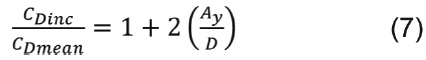Huera-Huarte and Bearman  more recently performed an experimental study using a vertical long flexible cylinder having a mass ratio of 1.8 with an external diameter of 16mm. They found that drag coefficients show larger values reaching values up to 3 than those calculated for stationary cylinders at the same Reynolds numbers. There is a clear amplification of drag as cross-flow amplitude increases. As the cross-flow response starts to reduce in amplitude and synchronization, the drag also reduces reaching values similar to those obtained for stationary cylinders at the same Reynolds numbers.

VIV Response Models
Current semi-empirical models for VIV response prediction highly depend on the experimentally derived values of hydrodynamic force coefficients. Therefore, most of those models rely on a large database of force coefficients. At low values of mass ratio, the response of a cylinder becomes highly influenced by the fluid. As a result, precise measurements are required in order to extract values of in-line drag forces which are influenced by cross-flow amplitudes. Many studies are based on the quasi-steady assumption which states that the response of a freely oscillating cylinder can be approximated to the response of a fixed cylinder under the action of oscillatory flow. As previously mentioned, at low values of mass ratio cross-flow amplitudes increase the values of mean drag coefficients and therefore accurate prediction of in-line drag coefficients are only possible when cross-flow amplitudes are considered. Konstantinidis , stated that it is difficult to infer the highly-complicated wake dynamics from flow fluctuations in the wake of transversely vibrating cylinder as a result it is recommendable to verify cross-flow amplitudes obtained from numerical simulations using experimental data. Other important fact noted by Konstantinidis  is that some discrepancies may be found in published data which call for further understanding of the complex nature of the VIV process. Therefore, numerical obtained cross-flow amplitudes are compared with cross-flow amplitudes obtained from the experimental model developed by Riveros et al. . A similar model is also used by Huera-Huarte and Bearman .

Governing Equations
The governing equations that will be employed for the proposed numerical model presented in this paper are the conservation law of mass and the conservation law of momentum as described in the following equations: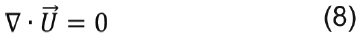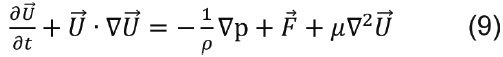where represents the total velocity vector, the body force per unit mass and p defined as the pressure. The finite volume method is used for the discretization of the conservation equations. The solution domain is then subdivided into a finite number of control volumes. The pressure correction scheme based on the SIMPLE algorithm is applied to couple the momentum equations with the continuity equations.

Numerical Implementation
The numerical implementation is carried out using the commercial finite volume CFD code, named FLUENT. Velocity inlet and outflow boundary conditions were used at inlet and outlet respectively. Symmetry boundary conditions are used to model the lower and upper limits of the computational domain as the expected pattern of the flow has mirror symmetry. The blockage ratio is defined as the cylinder diameter divided by the width of the solution domain, denoted as D/B. A higher blockage acts to decrease the rms value and the inertia coefficient of the in-line force exerted on the cylinder, and to increase the drag coefficient . Figure 1 depicts the computational domain. A blockage ratio (denoted as D/B) of 10% is selected in this paper based on the parametric study presented by Anagnostopoulos and Minear , who found that blockage effect is almost negligible for blockage ratios lower than 20%. The final grid is composed of 18450 nodes. The cylinder is placed in the center of a circular domain composed of triangular cells. The remaining regions of the computational domain are composed of quadrilateral cells. The solution is time-dependent. Therefore, an unsteady solver is used allowing the modeling of the oscillatory flow condition. The solution is time-dependent (time step of 0.01s). The numerical model is sinusoidally excited at Reynolds numbers up to 600 and Keulegan-Carpenter (KC) numbers up to 5, which correspond to a beta parameter of 120. Based on the Reynolds numbers achieved by the model, a laminar viscous model is selected. The magnitude of KC indicates different patterns of the vortex shedding at low values of KC, 1 < KC < 2, depending on β, the flow is symmetrical and remains attached to the cylinder. At KC ≈ 4, the flow separates but remains symmetrical as concentrations of vorticity are swept back over the cylinder when the flow reverses.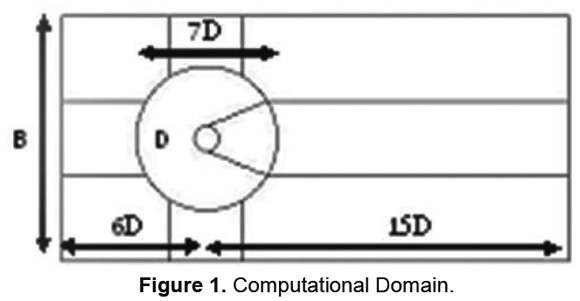The proposed model is first validated for steady flow at Re=100. Zhou and Graham , using a vortex-based method to simulate flow around a circular cylinder, found a value of CDmean of 1.37. They compared this value with 15 experimental and numerical results and found good agreement in their comparisons. The model proposed in this section is therefore implemented for the same simulation conditions presented by Zhou and Graham . The CDmean value computed from the proposed model is remarkably similar to the one presented by Zhou and Graham  having a value of 1.38. In order to compare with experimental values at different Re numbers, Figure 2 shows the CDmean values computed from the proposed model with the experimental values presented by Anderson .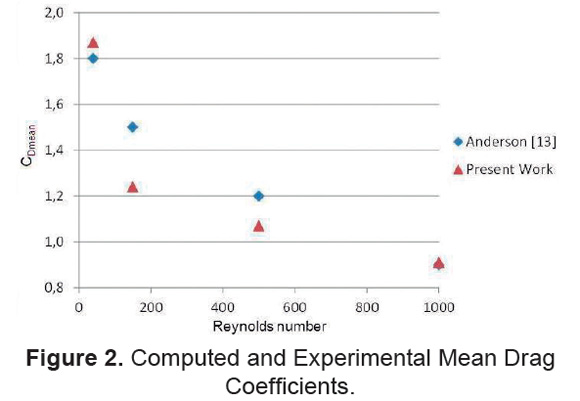It is then conducted the oscillatory flow simulation with amplitudes ranging from 0.0025 to 0.0125m and a period of 2s. These values correspond to KC values ranging from 1 to 4. Simulation results are then obtained at each time step. CDmean values are then calculated through least squares fit of the force time series. In order to perform a comparison with experimental data obtained at different levels of beta parameter, the experimental finding reported by Obasaju et al.  which states that there is a range of the beta parameter in which CDmean is not sensitive to a variation of beta parameter showing an upper boundary of beta parameter lies between 964 and 1204. Based on this statement, the results presented in this paper are compared with the ones found by Lin et al.  at a beta parameter of 70 as shown in Figure 3.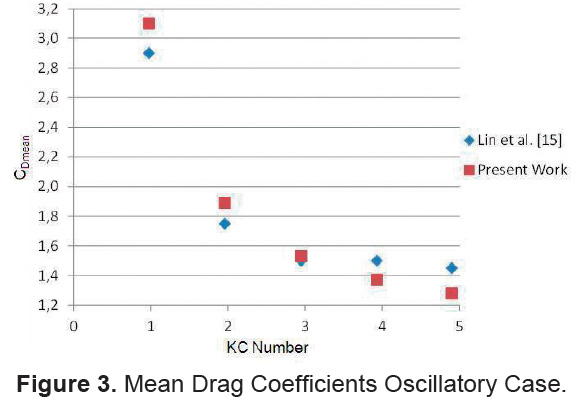Finally, the proposed CFD-based model is numerically implemented for the oscillating body case in order to represent the influence of cross-flow vibration influence on the drag force. The moving/deforming mesh capability provided by FLUENT is used herein to sinusoidally move the cylinder in the in-line direction while applying pressure forces in the cross-flow direction based on the explicit Euler formulation presented in Eq. 10 .The main idea of this procedure is to use the computed velocities at which the cylinder is excited in both in-line and cross-flow directions and then allows the cylinder to move in accordance with these velocities. In order to improve the simulation results, a time step of 0.001s. is selected in Eq. 10. By using this procedure it is possible to simulate the case of a cylinder with a low value of mass ratio.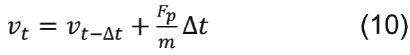where is the velocity of the cylinder in the cross-flow direction and is the pressure force in the same direction at a given value of time. The cylinder is then allowed to sinusoidally move in the streamwise direction with amplitudes ranging from 0.0025m to 0.0125m and period of 2s. In the cross-flow direction, the computed pressure forces are used to move the cylinder based on the cross-flow velocity computed from Eq. 10. The aforementioned procedure is repeated at each time step. Maximum cross-flow amplitudes were computed from the numerical model by performing a Fourier analysis and taking the maximum amplitude, the comparison with the experimental data provided by Riveros et al.  show good agreement. This comparison is supported on the statement made by Konstantinidis  that the comparison between forced excitation and free response is possible when the condition that the forced vibration replicates the motion of the cylinder in free vibration. It is important to note that it is recently demonstrated that for a cylinder excited transversely the added mass is also a function of the ratio of the cylinder's velocity to the velocity of the flow (added mass depends on both amplitude and frequency oscillation among other factors) . Due to the nonlinear nature of the cross-flow response for the results presented in this paper the maximum amplitude obtained from the Fourier spectrum is selected. Further information related to the type of in-line and cross-flow responses can be found in Riveros et al. . It is important to note that it is highly nonlinear exhibiting a very complex response and it is not possible to clearly define the sheading frequency. Figure 4 shows predicted CDinc using the empirical formulation derived by Vandivier  as shown in Eq. 1, the empirical formulation derived by Sarpkaya  as shown in Eq. 12 and the computed values obtained from the oscillating case. CDmean was obtained from the oscillatory case results in order to use the aforementioned equations.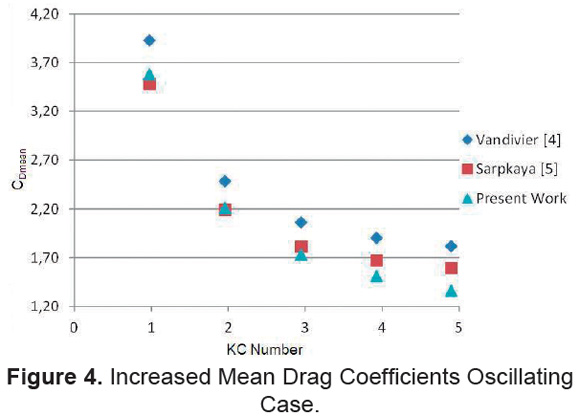Results and Discussion

It is possible to observe from Figure 4 that allowing the cylinder to freely move in the cross-flow direction produces an increment of the drag force. The prediction of the increment of the drag force as previously mentioned is a function of the cross-flow amplitude and therefore accurate prediction is still challenging due to the nonlinear nature of the VIV. Good agreement is observed between the prediction using the empirical formulation derived by Sarpkaya  and the numerical results presented in this paper. The maximum error between Eq. 1 and the results presented in this paper is 15%. Good agreement is observed at low KC numbers and the maximum deviation is presented at KC=5. The simulation results presented in Figure 5 show that it is important to consider the value of the beta parameter in the calculation of the inertia coefficient (Cm). It is also important to highlight that for the oscillating body case the added-mass coefficient Ci is obtained through least squares fit of the force time series and it is assumed that Cm=Ci+1.0. Finally, it is observed that there is no significant variation of Cm for the KC numbers presented in this paper. The computed values of Cm show lower values than the ones obtained from the oscillatory flow case showing the importance of fluid-structure interaction, which is expected to be significant at large amplitudes.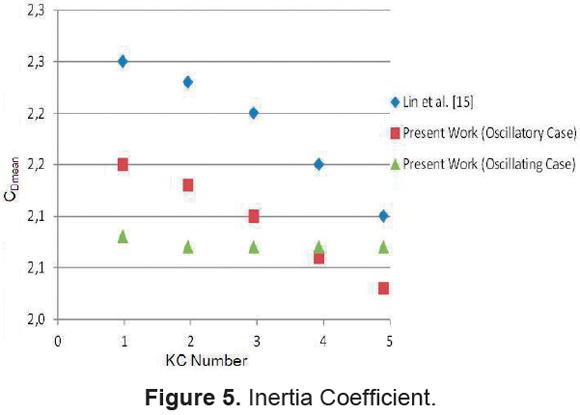Conclusions

Based on the simulation results obtained from the CFD-based model presented in this paper, it is possible to observe that the inertia coefficient depends on the beta parameter for the range of the KC numbers presented in this paper. On the other hand, the mean drag coefficient for oscillating cylinders can be indistinctly obtained from the oscillatory flow case without much incidence on the final simulation results as compared with the oscillating case. Further studies are needed in order to develop a CFD-based model for oscillating bodies. It is important to highlight that the quasi-steady assumption neglects fluid-structure interaction and therefore introduces a source of error in the calculation of the hydrodynamic force coefficients at large amplitude response. It is still challenging the numerical prediction of the increment in drag forces due to transverse oscillations, the numerical model presented in this paper was developed in order to kinematically represent an experimental model and therefore cross-flow amplitudes were verified before conducting the comparison with semi-empirical formulations. Mass ratio which is not considered in this study may play an important role leading to larger amplitudes as it increases. This aspect must be relevant due to the fact that lock-in events are inherently related to how the different frequencies interact, i.e. frequency of vortex shedding, natural frequency.

Acknowledgements

This work was supported by CODI - University of Antioquia under Project MC10-1-04.

References

 Sarpkaya T. Hydrodynamic damping, flow-induced oscillations and biharmonic response. Journal of Offshore Mechanics and Artic Engineering ASME. 1995;117:232-8.         [ Links ]

 Kim W, Perkins N. Coupled slow and fast dynamics of flow excited elastic cable systems. Journal of Vibration and Acoustics. 2003; 125:155-61.         [ Links ]

 Bishop R, Hassan A. The lift and drag forces on a circular cylinder oscillating in a flowing flow. En: Proceedings of the Royal Society of London. Series A: Mathematical and Physical Science; 1964 January 7; London, England. London: the Royal Society of London;1964. p. 32-50.         [ Links ]

 Vandivier J. Drag coefficients of long flexible cylinders. En: Offshore Technology Conference; 1983 May 2-5; Houston, The United States. Houston: Offshore Technology Conference; 1983. p. 25-30.         [ Links ]

 Sarpkaya T. Fluid forces on oscillating cylinders. ASCE Journal of Waterway, Port, Coastal, and Ocean Division. 1978;104:275- 90.         [ Links ]

 Chaplin JR, Bearman PW, Cheng Y, Fontaine E, Graham JMR, Herfjord M, et al. Blind predictions of laboratory measurements of vortex-induced vibrations of a tension riser. Journal of Fluids and Structures. 2005;21:25- 40.         [ Links ]

 Khalak A, Williamson CHK. Motions, forces and mode transitions in vortex-induced vibrations at low mass-damping. Journal of Fluids and Structures. 1999;13:813-51.         [ Links ]

 Willden RHJ, Graham JMR. Three-distinct response regimes for the transverse vortex-induced vibrations of circular cylinders at low Reynolds numbers. Journal of Fluids and Structures. 2006;22:885-95.         [ Links ]

 Huera-Huarte FJ, Bearman PW. Wake structures and vortex-induced vibrations of a long flexible cylinder - Part 2: Drag coefficients and vortex modes. Journal of Fluids and Structures. 2009;25:991-1006.         [ Links ]

 Konstantinidis E. On the response and wake modes of a cylinder undergoing streamwise vortex-induced vibration. Journal of Fluids and Structures. 2014;45:256-62.         [ Links ]

 Riveros CA, Utsunomiya T, Maeda K, Kazuaki I. Response prediction of long flexible risers subject to forced harmonic vibration. Journal of Marine Science and Technology. 2010;15:44- 53.         [ Links ]

 Anagnostopoulos P, Minear R. Blockage effect of oscillatory flow past a fixed cylinder. Applied Ocean Research. 2004;26:147-53.         [ Links ]

 Zhou C, Graham J. A numerical study of cylinders in waves and currents. Journal of Fluids and Structures. 2000;14:403-28.         [ Links ]

 Anderson JD. Fundamentals of Aerodynamics. New York: McGraw-Hill; 1991.         [ Links ]

 Obasaju ED, Bearman PW, Graham JMR. A study of forces, circulation and vortex patterns around a circular cylinder in oscillating flow. Journal of Fluid Mechanics. 1988;196:467-94.         [ Links ]

 Lin X.W, Bearman PW, Graham JMR. A numerical study of oscillatory flow about a circular cylinder for low values of beta parameter. Journal of Fluids and Structures. 1996;10:501-26.         [ Links ]

 ANSYS FLUENT. ANSYS Inc; 2011.         [ Links ]

 Riveros CA. Response prediction and damage assessment of flexible risers (doctoral thesis). Kyoto, Japan: University; 2008.         [ Links ]